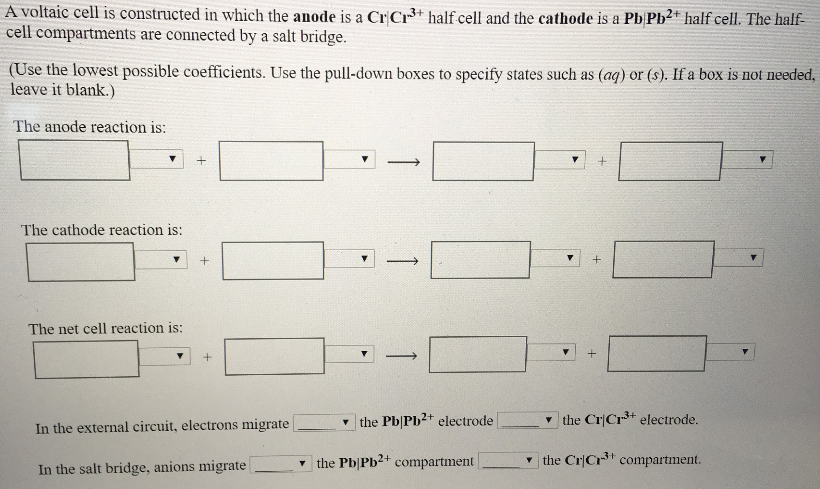# A voltaic cell is constructed in which the anode is a Cr|Cr^3+ half cell and the cathode is a Pb|Pb^2+ half cell. The half-cell compartments are connected by a salt bridge. (Use the lowest possible coefficients. Use the pull-down boxes to specify states such as (aq)) or (s). If a box is not needed, leave it blank.) The anode reaction is: The cathode reaction is: The net cell reaction is: In the external circuit, electrons migrate ____ the Pb|Pb^2+ electrode ____ the Cr|Cr^3+ electrode. In the salt bridge, anions migrate ____ the Pb|Pb^2+ compartment ____ the Cr|Cr^3+ compartment.